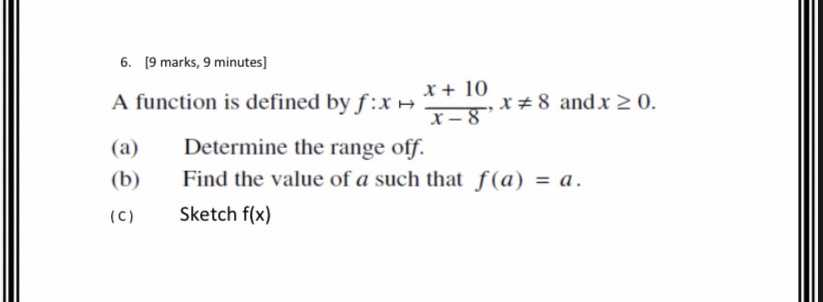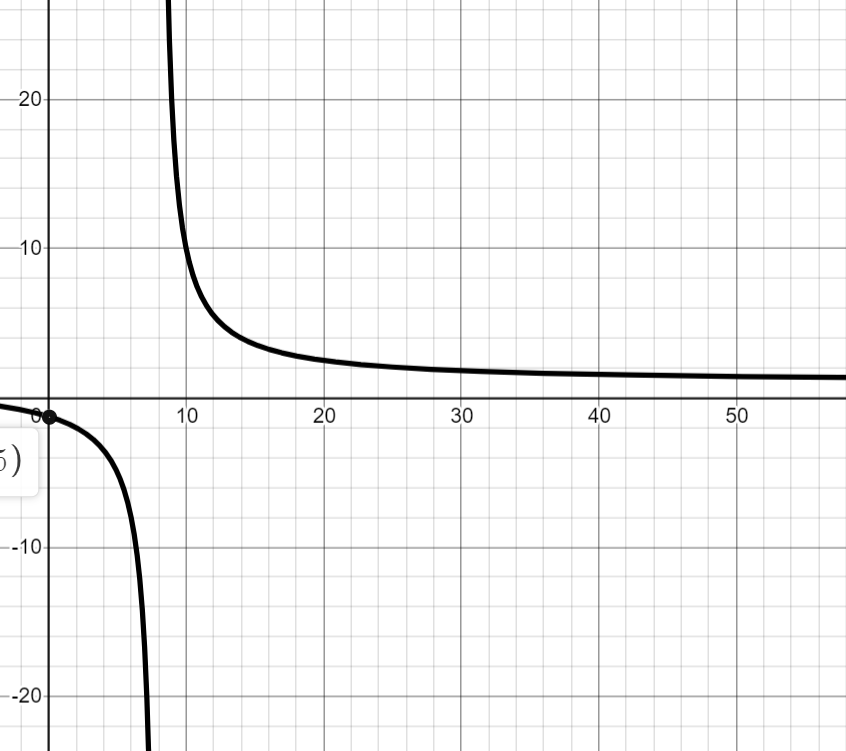### ¿Todavía tienes preguntas de matemáticas?

Pregunte a nuestros tutores expertos
Algebra
PreguntaA function is defined by $$f : x \mapsto \frac { x + 10 } { x - 8 } , x \neq 8$$ and $$x \geq 0$$ .

(a) Determine the range of $$f$$ .

(b) Find the value of $$a$$ such that $$f ( a ) = a$$

(c) Sketch $$f ( x )$$

c.